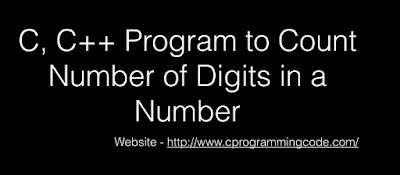# Programming Videos

## Search

### C, C++ Program to Count Number of Digits in a NumberC, C++ Program to Count Number of Digits in a Number
Write a C, C++ program to count number of digits in a number. Given a positive input number, Our program will count the number of digits present in a number. Suppose a user has entered a number 4567, So the total digits in a number is 4.

## Logic to Count Number of Digits in a Number

C, C++ Interview Questions

Program to reverse a number

Program to Find the sum of first n odd numbers

## C Program to Count Number of Digits of a Number

```#include <stdio.h>

int main() {

int num;
int count=0;

printf("Enter a number \n");
scanf("%d", &num);

/* If a number is greater than zero. */

while(num > 0) {

/* Reduce the number is every iteration. */

num=num/10;

/* Increment the count. */

count++;
}

printf("Number of digits in a number is %d",count);

return 0;
}

```

Output:

Enter a number - 4567

Number of digits in a number is - 4

## C++ Program to Count Number of Digits in a Number

```#include<iostream>
using namespace std;

int main() {

int num, count = 0;

// Input number
cout << "Enter a number \n";
cin  >> num;

// If number is greater than zero
while (num > 0) {

//Reduce number in each iteration
num = num / 10;

//Count number of digits
count++;
}

cout << "Number of digits in a number is " << count;

return 0;
}```